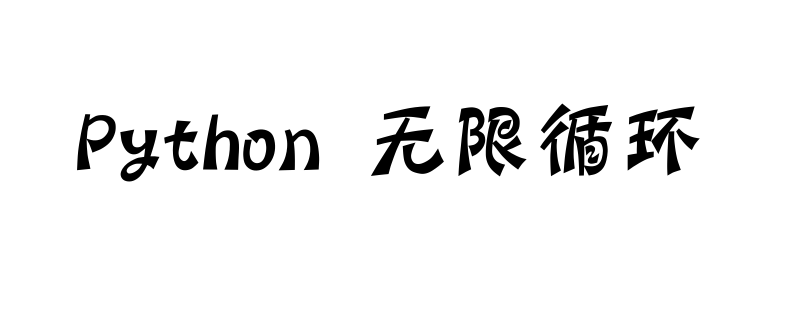• 首页
• 更多

# Python中如何解决无限循环的问题```while True:
print("hello")```

```while True:
s = input("请输入内容")
if(s):
print(s, "输入的文字")```

```l = 

for i in l:
print(i)
l.append(i+1)```

```while True:
print("hello")```

```i = 0
while True:
print("hello")
i += 1
if (i ==10):
break```

```while True:
s = input("请输入内容")
if(s):
print(s, "输入的文字")```

```while True:
s = input("请输入内容")
if(s):
print(s, "输入的文字")
else:
break```

```l = 

for i in l:
print(i)
l.append(i+1)```

```l = [0, 1, 2, 3, 4]

for i in l:
print(i)```

• 原生JS实现别踩白块小游戏（九）
• python中运算符怎么使用
• 防火墙是什么意思？有什么用？
• Unix是什么
• 原生JS实现别踩白块小游戏（八）